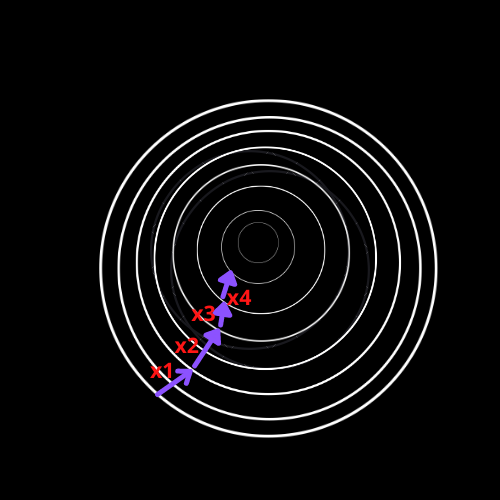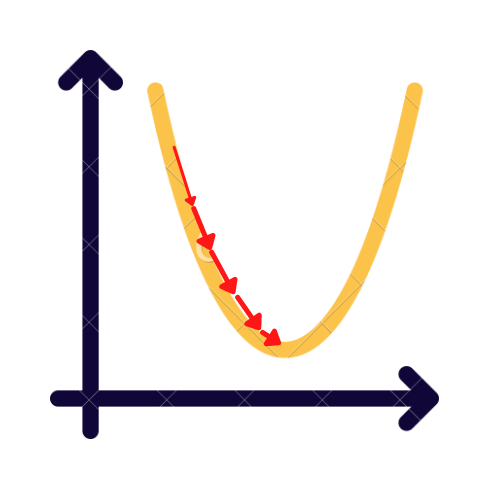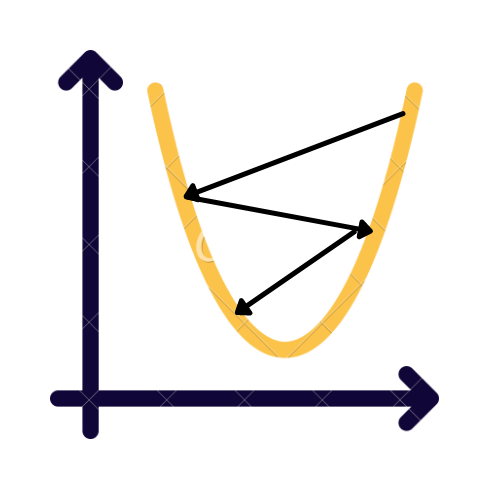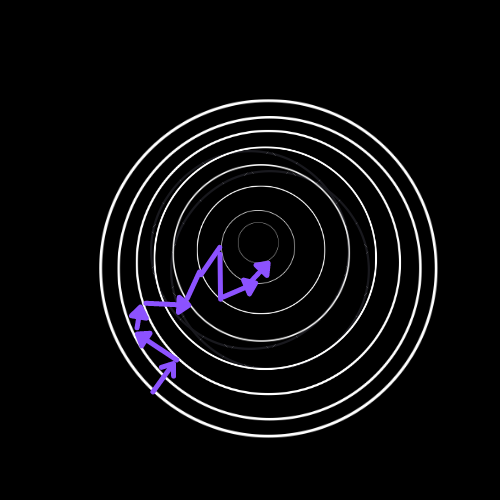## Introduction

Gradient Descent is one of the most helpful optimization techniques used in Machine Learning and Deep Learning. Gradient means the slope of the surface or, in mathematics, a function,i.e., rate of change of a variable concerning another variable.

There are three types of gradient descent known:

So basically, Gradient Descent is an iterative algorithm that starts from a random point of the curve and descends or decreases down the slope until it reaches the optimal minimum value of a function. When the slope is far from zero, we should take significant steps, and baby steps are taken closer to zero. The steeper slope means the model will learn faster, but when slopes become zero, the model stops learning. The length of steps taken depends upon a factor known as the learning rate.One of the primary use cases of Gradient Descent is Linear Regression, in which we try to minimize the loss function,i.e., to minimize the squared residuals.

## Learning Rate

A Learning rate is a randomly chosen number that tells the step's size. The Gradient Descent algorithm multiplies the Gradient by the learning rate to determine the next point. A small learning rate will take forever to reach the minima known as vanishing gradient descent, and a significant learning rate will skip the minima known as exploding gradient descent.Small Learning RateBig Learning Rate

Determining the learning rate value is very troublesome as it can vanish or explode. Some of the shared values of the learning rate chosen manually are 0.1, 0.001, 0.0001. Specific methods, such as AdaGram and RMSProp methods, determine the most fitting learning rate.

The Gradient Descent algorithm has a few drawbacks, making it computationally expensive. Let's dig deeper into the issue. Suppose we have 1,00,000 data points and ten features. Thus, when we calculate the loss function, the sum of squared residuals of every data point, we compute the function's derivative concerning each feature will be doing 10*1,00,000 computations per iteration. Let's consider it will be doing 1000 iterations, so in total, it will be 100 million computations to reach the minimum, which is so expensive.

So to solve this issue, Stochastic Gradient Descent came into the picture.

Stochastic, in simpler terms, means random. Stochastic Gradient Descent is used for big data sets where Gradient Descent can be computationally expensive. In Stochastic Gradient Descent, we approximate the Gradient using only one randomly selected data point, which is computationally cheap compared to the Gradient Descent.

Thus, the Gradient of the cost function is calculated for each component separately, not for all elements in the sample, as done with the traditional gradient descent method. The Gradient calculated for a particular element approximates the actual Gradient.

In Stochastic Gradient Descent, since we choose only one randomly selected data point from the dataset, the path taken by the SGD to reach the minima is usually not optimal as accepted by the Gradient Descent algorithm. But the path taken by the SGD or GD doesn't matter that much as long as we reach the most optimal value in minimum time.

Due to its randomness, this algorithm is more irregular or noise increases. Due to its randomness, the SGD algorithm never settles down. There is always a movement, so the final parameter achieved may not be the most optimal, but it's good.One advantage of SGD randomness is that it jumps out of the local minima when the cost function is too irregular. In an irregular cost function, Stochastic Gradient Descent has the advantage over Batch Gradient Descent.

But one drawback is that it never settles down, which doesn't provide the most optimal minima. One solution to this drawback is to decrease the learning rate gradually. At first, we take significant steps to escape the local minima. Still, when it's near zero, baby steps are taken, allowing the algorithm to settle down at the global minima or the most optimal value.

• Shuffle the dataset randomly.
• Choose a data point randomly and cycle through all the elements.
• Cycle on all weights or parameters.
• Adjust the current weight or parameter under the derivative of the cost function.
• Calculate the new Gradient with the new value of the parameter or weights.
• Repeat all the above steps until convergence or global minima is achieved.

The Pseudocode below shows the Stochastic Gradient Descent algorithm function.

SGD vs. GD

The main difference between SGD and GD is the time taken for each iteration which is low in the case of SGD and high for GD. The number of points traversed before updating parameters is 1 in the case of SGD and the input data size in the case of GD. Because of this few things happen:

• The computation is fast, but convergence is slow in SGD. Because the Gradient we get in SGD is just an approximation of the actual Gradient, we are not taking all the data points for computing the Gradient whereas, in GD, the Gradient we obtain is the actual Gradient. So in GD, we always move in descending Gradient to achieve faster convergence.

• SGD is too sensitive to outliers. While randomly picking a data point, if we encounter an outlier, it will deviate from its standard path and take some extra updates to get back on its track of convergence.

• In SGD, the chances of overfitting are less. Overfitting happens when the model repeatedly considers the same data points, such that its parameters are adjusted according to only those data points (instead of learning the pattern). So for the test data sets, the error rate is high. But in SGD, we chose different data points at random, restricting the model from learning the answer. Thus, we can say SGD is less prone to overfitting.

We usually don't use GD alone because it is slow. Adam and momentum-based SGD are the most commonly used optimizers.

1. Does Stochastic Gradient Descent always converge?
Ans. Stochastic Gradient Descent helps find the optimal points, but these optimal points are not necessarily global. Due to its randomness, the step size might be too large to escape the optimal point. There is always a movement, so the final parameter achieved may not be optimal; hence it may not converge.

2. What is the intuition behind Gradient Descent?
Ans. Gradient descent is an optimization algorithm used on a convex function to achieve the local minima,i.e., when slope equals zero. After every iteration, the parameters have to be updated so that the slope moves towards the local minima. The parameters or weights are being updated with the help of a parameter called the learning rate. As the algorithm is too sensitive to the learning rate, we should be careful in determining its value,i.e., it should be neither too small nor too large.

3. What is the difference between GD and SGD?
Ans. The main difference between SGD and GD is the time taken for each iteration which is low in the case of SGD and high for GD, whereas the convergence is slow in the case of SGD.

Ans. Gradient descent fails when the function is not differentiable because we can't update the parameters at that point.

## Key Takeaways

So that's the end of the article. Let's brief out the article:

This article explains the in-depth intuition behind Gradient Descent and the need to improve our algorithm to make it computationally less expensive.

We have discussed Stochastic Gradient Descent, its certain advantages, and a few drawbacks.

Further, we discussed learning rates and how GD and SGD are sensitive to them.

And at last, we discuss the fundamental differences between GD and SGD.

Isn't Machine Learning exciting!! We build different models using different algorithms and improve those algorithms to achieve higher accuracy. So are you excited to learn different machine learning algorithms, don't be confused; we have a perfect partner, the MACHINE LEARNING COURSE, to guide you in your learning process.

Happy Learning Ninjas!!